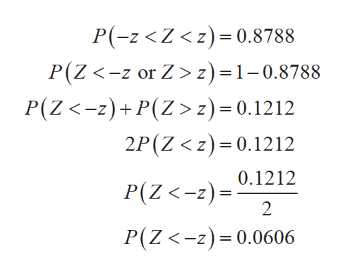# Let Z be the standard normal variable. Find z if P(-z < Z < z) = 0.8788. (Give your answer to two decimal places.)

Question
2 views

Let Z be the standard normal variable. Find z if P(-z < Z < z) = 0.8788. (Give your answer to two decimal places.)

check_circle

Step 1

The value of z is cal...help_outlineImage TranscriptioncloseP(-z z) =1-0.8788 P(Z-2)P(Z>z)= 0.1212 2P(Z < z) 0.1212 0.1212 P(Z <-z)= 2 P(Z<-z) 0.0606 fullscreen

### Want to see the full answer?

See Solution

#### Want to see this answer and more?

Solutions are written by subject experts who are available 24/7. Questions are typically answered within 1 hour.*

See Solution
*Response times may vary by subject and question.
Tagged in

### Other